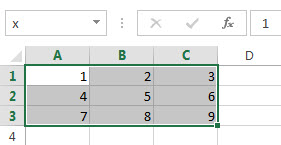## Return Multiple Output Arguments from MATLAB Function

This example shows how to execute a MATLAB® function that returns multiple output arguments in Microsoft® Excel® using a Microsoft Excel VBA macro. The macro writes multiple output arguments from the MATLAB workspace to Microsoft Excel cells.

This example calculates the singular value decomposition of a matrix using `svd`.

In the Microsoft Excel cells from A1 through C3, create a range of data. Enter numbers from 1 through 3 in cells A1 through A3. Enter numbers from 4 through 6 in cells B1 through B3. Enter numbers from 7 through 9 in cells C1 through C3.Create a Microsoft Excel VBA macro named `applysvd`. For details about creating macros, see Excel Help.

```Public Sub applysvd() MLOpen MLPutMatrix "x", Range("A1:C3") MLEvalString ("[u,s,v] = svd(x);") MLGetMatrix "u", "A5" MLGetMatrix "s", "A9" MLGetMatrix "v", "A13" MatlabRequest MLClose End Sub```

The macro:

1. Starts MATLAB.

2. Sends the data in the A1 through C3 cell range to the MATLAB workspace and assigns it to the MATLAB variable `x`.

3. Runs `svd` with the input argument `x` and output arguments `u`, `s`, and `v`.

4. Individually retrieves data for one output argument into a specific Microsoft Excel cell while accounting for the size of each output data matrix to avoid overwriting data. For the first output argument, the macro retrieves the data for the output argument `u` into cell A5.

5. Closes MATLAB.

Run `applysvd`. MATLAB runs `svd` and populates the specified cells with data from the three output arguments.For details about running macros, see Excel Help.